## How do I draw a vertical text in Java 2D?

To draw a text / string vertically we need to do a transform on the `Graphics2D` object. First, create an instance of `AffineTransform` and set the rotation using the `setToRotation()` method. And then pass this transform object into `g2.setTransform()` method.

``````package org.kodejava.example.geom;

import javax.swing.*;
import java.awt.*;
import java.awt.geom.AffineTransform;

public class DrawVerticalText extends JPanel {
@Override
public void paint(Graphics g) {
Graphics2D g2 = (Graphics2D) g;

// Define rendering hint, font name, font style and font size
g2.setRenderingHint(RenderingHints.KEY_ANTIALIASING, RenderingHints.VALUE_ANTIALIAS_ON);
g2.setFont(new Font("Segoe Script", Font.BOLD, 22));
g2.setColor(Color.RED);

// Rotate 90 degree to make a vertical text
AffineTransform at = new AffineTransform();
at.setToRotation(Math.toRadians(90), 80, 100);
g2.setTransform(at);
g2.drawString("This is a vertical text", 10, 10);
}

public static void main(String[] args) {
JFrame frame = new JFrame();
frame.setTitle("Draw Vertical Text Demo");
frame.setDefaultCloseOperation(WindowConstants.EXIT_ON_CLOSE);
frame.add(new DrawVerticalText());
frame.pack();
frame.setSize(420, 350);
frame.setVisible(true);
}
}
``````

Run the snippet and you’ll see the following screen: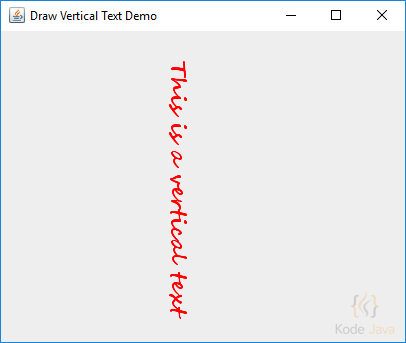## How do I draw a string in Java 2D?

The code snippet below show you how to draw a string using `Graphics2D`. The `drawString()` method accept the string to be drawn and their `x` and `y` coordinate. Here you can also see how to set the antialiasing mode using the `setRenderingHint()` method.

``````package org.kodejava.example.geom;

import javax.swing.*;
import java.awt.*;

public class DrawString extends JPanel {
@Override
public void paint(Graphics g) {
Graphics2D g2 = (Graphics2D) g;

// Define rendering hint, font name, font style and font size
g2.setRenderingHint(RenderingHints.KEY_ANTIALIASING, RenderingHints.VALUE_ANTIALIAS_ON);
g2.setFont(new Font("Segoe Script", Font.BOLD + Font.ITALIC, 40));
g2.setPaint(Color.ORANGE);

// Draw Hello World String
g2.drawString("Hello World!", 50, 100);
}

public static void main(String[] args) {
JFrame frame = new JFrame();
frame.setTitle("Draw String Demo");
frame.setDefaultCloseOperation(WindowConstants.EXIT_ON_CLOSE);
frame.add(new DrawString());
frame.pack();
frame.setSize(420, 300);
frame.setVisible(true);
}
}
``````

Run the snippet and you’ll see the following screen:## How do I create a gradient paint in Java 2D?

To change the color of a graphics shape we can use the `setPaint()` method. For a simple coloring we can pass the color object into this method, such as `Color.RED` or `Color.GREEN`.

If you want to paint with a gradient paint you can use the GradientPaint class. This class provides a way to fill a shape with a linear color gradient pattern. To create a gradient color pattern you can pass the following parameter to the object constructor.

• `x1`: x coordinate of the first specified point in user space
• `y1`: y coordinate of the first specified point in user space
• `color1`: color at the first specified point
• `x2`: x coordinate of the second specified point in user space
• `y2`: y coordinate of the second specified point in user space
• `color2`: color at the second specified point
``````package org.kodejava.example.geom;

import javax.swing.*;
import java.awt.*;
import java.awt.geom.Rectangle2D;

public class GradientPaintDemo extends JComponent {
@Override
public void paint(Graphics g) {
Graphics2D g2 = (Graphics2D) g;

GradientPaint blackToGray = new GradientPaint(50, 50, Color.BLACK,
300, 100, Color.LIGHT_GRAY);
g2.setPaint(blackToGray);
g2.fill(new Rectangle2D.Double(50, 50, 300, 100));

GradientPaint blueToBlack = new GradientPaint(0, 0, Color.BLUE,
400, 400, Color.BLACK);
g2.setPaint(blueToBlack);
g2.fill(new Rectangle2D.Double(50, 160, 300, 100));
}

public static void main(String[] args) {
JFrame frame = new JFrame("Gradient Paint Demo");
frame.setDefaultCloseOperation(WindowConstants.EXIT_ON_CLOSE);
frame.getContentPane().add(new GradientPaintDemo());
frame.pack();
frame.setSize(new Dimension(420, 350));
frame.setVisible(true);
}
}
``````

This code snippet produce the following output: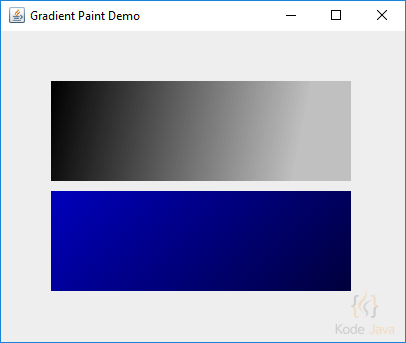## How do I define stroke when drawing a shape in Java 2D?

``````package org.kodejava.example.geom;

import javax.swing.*;
import java.awt.*;
import java.awt.geom.Line2D;

public class DrawStrokeDemo extends JComponent {
@Override
public void paint(Graphics g) {
Graphics2D g2 = (Graphics2D) g;

float[] strokes = {1, 2, 3, 4, 5, 6};
for (float stroke : strokes) {
g2.setStroke(new BasicStroke(stroke));
g2.draw(new Line2D.Float(50, stroke * 20, 350, stroke * 20));
}
}

public static void main(String[] args) {
JFrame frame = new JFrame("Draw Stroke Demo");
frame.setDefaultCloseOperation(WindowConstants.EXIT_ON_CLOSE);
frame.getContentPane().add(new DrawStrokeDemo());
frame.pack();
frame.setSize(new Dimension(420, 200));
frame.setVisible(true);
}
}
``````

This code snippet produce the following output: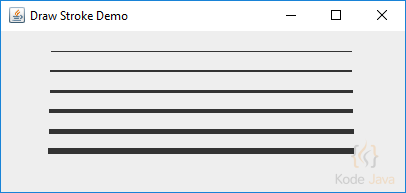## How do I create a dashed stroke in Java 2D?

``````package org.kodejava.example.geom;

import javax.swing.*;
import java.awt.*;
import java.awt.geom.RoundRectangle2D;

public class DrawDashedStroke extends JComponent {
@Override
public void paint(Graphics g) {
Graphics2D g2 = (Graphics2D) g;
float[] dash = {10.0f, 5.0f, 3.0f};

// Creates a dashed stroke
Stroke dashed = new BasicStroke(2.0f, BasicStroke.CAP_BUTT,
BasicStroke.JOIN_MITER, 10.0f, dash, 0.0f);

g2.setStroke(dashed);
g2.setPaint(Color.RED);
g2.draw(new RoundRectangle2D.Double(50, 50, 300, 100, 10, 10));
}

public static void main(String[] args) {
JFrame frame = new JFrame("Draw Dashed Stroke Demo");
frame.setDefaultCloseOperation(WindowConstants.EXIT_ON_CLOSE);
frame.getContentPane().add(new DrawDashedStroke());
frame.pack();
frame.setSize(new Dimension(420, 250));
frame.setVisible(true);
}
}
``````

This code snippet produce the following output:## How do I draw an arc in Java 2D?

`Arc2D` is the abstract superclass for all objects that store a 2D arc defined by a framing rectangle, start angle, angular extent (length of the arc), and a closure type (`Arc2D.OPEN`, `Arc2D.CHORD`, or `Arc2D.PIE`).

To constructs a new arc in double values, such as defining the specified location, size, angular extents, and closure type we can use the `Arc2D.Double` static-inner class.

``````package org.kodejava.example.geom;

import javax.swing.*;
import java.awt.*;
import java.awt.geom.Arc2D;

public class DrawArc extends JComponent {
@Override
public void paint(Graphics g) {
Graphics2D g2 = (Graphics2D) g;

g2.setStroke(new BasicStroke(2.0f));
g2.setPaint(Color.RED);
g2.draw(new Arc2D.Double(50, 50, 300, 300, 0, 90, Arc2D.PIE));
g2.setPaint(Color.GREEN);
g2.draw(new Arc2D.Double(50, 50, 300, 300, 90, 90, Arc2D.PIE));
g2.setPaint(Color.BLUE);
g2.fill(new Arc2D.Double(50, 50, 300, 300, 180, 90, Arc2D.PIE));
}

public static void main(String[] args) {
JFrame frame = new JFrame("Draw Arc Demo");
frame.setDefaultCloseOperation(WindowConstants.EXIT_ON_CLOSE);
frame.getContentPane().add(new DrawArc());
frame.pack();
frame.setSize(new Dimension(420, 450));
frame.setVisible(true);
}
}
``````

Here is the result you’ll get when you run the snippet:## How do I draw an ellipse in Java 2D?

The `Ellipse2D` class define an ellipse that is defined by a framing rectangle. You can create an ellipse using a double or float values. When creating an ellipse using double values use the `Ellipse2D.Double` class. And for the float values you can use the `Ellipse2D.Float` class.

``````package org.kodejava.example.geom;

import javax.swing.*;
import java.awt.*;
import java.awt.geom.Ellipse2D;

public class DrawEllipse extends JComponent {
@Override
public void paint(Graphics g) {
Graphics2D g2 = (Graphics2D) g;

g2.setPaint(Color.RED);
g2.setStroke(new BasicStroke(5.0f));
g2.draw(new Ellipse2D.Double(50, 50, 250, 250));

g2.setPaint(Color.BLUE);
g2.fill(new Ellipse2D.Double(10, 10, 40, 40));

g2.setPaint(Color.YELLOW);
g2.fill(new Ellipse2D.Double(10, 300, 40, 40));

g2.setPaint(Color.GREEN);
g2.fill(new Ellipse2D.Double(300, 300, 40, 40));

g2.setPaint(Color.ORANGE);
g2.fill(new Ellipse2D.Double(300, 10, 40, 40));
}

public static void main(String[] args) {
JFrame frame = new JFrame("Draw Ellipse Demo");
frame.setDefaultCloseOperation(WindowConstants.EXIT_ON_CLOSE);
frame.getContentPane().add(new DrawEllipse());
frame.pack();
frame.setSize(new Dimension(420, 400));
frame.setVisible(true);
}
}
``````

Here is the result you’ll get when you run the snippet:## How do I draw a GeneralPath in Java 2D?

``````package org.kodejava.example.geom;

import javax.swing.*;
import java.awt.*;
import java.awt.geom.GeneralPath;

public class DrawGeneralPath extends JComponent {
@Override
public void paint(Graphics g) {
Graphics2D g2 = (Graphics2D) g;

g2.setStroke(new BasicStroke(4.0f));
g2.setPaint(Color.GREEN);

int[] xPoints = {10, 50, 100, 150, 200, 250, 300, 350};
int[] yPoints = {10, 50,  10,  50,  10,  50,  10,  50};
GeneralPath path = new GeneralPath(GeneralPath.WIND_EVEN_ODD,
xPoints.length);

// Adds point to the path by moving to the specified
// coordinates.
path.moveTo(xPoints, yPoints);
for (int i = 1; i < xPoints.length; i++) {
// Adds a point to the path by drawing a straight
// line from the current position to the specified
// coordinates.
path.lineTo(xPoints[i], yPoints[i]);
}
path.curveTo(150, 150, 300, 300, 50, 250);
path.closePath();
g2.draw(path);

// Draw another path, a start
g2.setPaint(Color.RED);
g2.setStroke(new BasicStroke(2.0f));
path = new GeneralPath(GeneralPath.WIND_NON_ZERO);
path.moveTo(200, 50);
path.lineTo(270, 300);
path.lineTo(100, 120);
path.lineTo(300, 120);
path.lineTo(130, 300);
path.closePath();
g2.draw(path);

}

public static void main(String[] args) {
JFrame frame = new JFrame("Draw GeneralPath Demo");
frame.setDefaultCloseOperation(WindowConstants.EXIT_ON_CLOSE);
frame.getContentPane().add(new DrawGeneralPath());
frame.pack();
frame.setSize(new Dimension(420, 400));
frame.setVisible(true);
}
}
``````

Here is the result you’ll get when you run the snippet: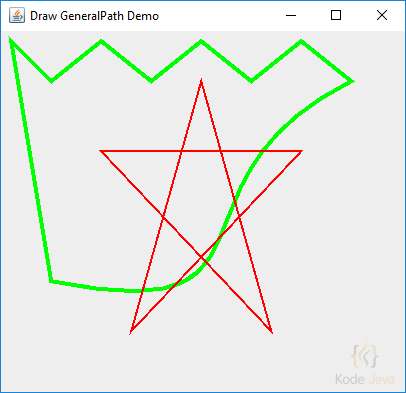## How do I draw a round rectangle in Java 2D?

The `RoundRectangle2D` class defines a rectangle with rounded corners defined by a location `(x,y)`, dimension `(w x h)`, and the width and height of an arc with which to round the corners.

The `RoundRectangle2D.Double` class constructs a `RoundRectangle2D` from the specified values in double, including the location, width and the arch of the round rectangle.

``````package org.kodejava.example.geom;

import javax.swing.*;
import java.awt.*;
import java.awt.geom.RoundRectangle2D;

public class DrawRoundRectangle extends JComponent {
@Override
public void paint(Graphics g) {
Graphics2D g2 = (Graphics2D) g;

g2.setPaint(Color.RED);
g2.setStroke(new BasicStroke(2.0f));

double x = 50;
double y = 50;
double w = x + 250;
double h = y + 100;
g2.draw(new RoundRectangle2D.Double(x, y, w, h, 50, 50));
}

public static void main(String[] args) {
JFrame frame = new JFrame("Rounded Rectangle Demo");
frame.setDefaultCloseOperation(WindowConstants.EXIT_ON_CLOSE);
frame.getContentPane().add(new DrawRoundRectangle(), BorderLayout.CENTER);
frame.pack();
frame.setSize(420, 300);
frame.setVisible(true);
}
}
``````

Here is the result you’ll get when you run the snippet: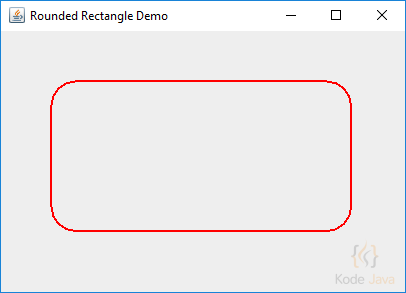## How do I draw a rectangle in Java 2D?

The code snippet below show you how to use the `Graphics2D` class the draw a rectangle. You can see the snippet in the `paintComponent(Graphics g)` method defined in the anonymous `JPanel` object.

To draw a rectangle we use the `Rectangle2D.Double` static-inner class. The constructor of this class accept the information about the rectangle `x`, `y` coordinates and its `width` and `height`.

``````package org.kodejava.example.awt.geom;

import javax.swing.*;
import java.awt.*;
import java.awt.geom.Rectangle2D;

public class DrawRectangle {
public static void main(String[] args) {
SwingUtilities.invokeLater(new Runnable() {
@Override
public void run() {
JFrame frame = new JFrame("Draw Rectangle");
frame.setDefaultCloseOperation(WindowConstants.EXIT_ON_CLOSE);
frame.add(new JPanel() {
@Override
protected void paintComponent(Graphics g) {
// Draw a rectangle using Rectangle2D class
Graphics2D g2 = (Graphics2D) g;
g2.setColor(Color.RED);

double x = 100;
double y = 100;
double width = x + 200;
double height = y + 50;

// Draw the red rectangle
g2.draw(new Rectangle2D.Double(x, y, width, height));

g2.setColor(Color.BLUE);
// Draw the blue rectangle
g2.draw(new Rectangle2D.Double(150, 50, 200, 250));
}
}, BorderLayout.CENTER);

frame.pack();
frame.setSize(new Dimension(500, 400));
frame.setVisible(true);
}
});
}
}
``````

Here is the result you’ll get when you run the snippet: